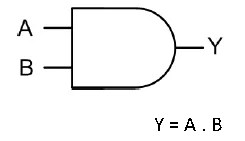How to make an AND Gate using Diodes on BreadboardI am sure you must be familiar with an AND Gate, it’s Truth Table, Logic symbol, and its working. But do you know, you can build your own And Gate on Breadboard?  So in this post, you will learn how to make an AND Gate using Diodes on Breadboard.

What is an AND Gate?And gate is a Logic Gate and called so because AND means “to multiply”. There are two inputs and one output in an AND Gate.  So only when both inputs are one, the output is one and zero in all the other cases.

You can also watch the video below for quick reference:

Truth Table:

Components required:

1. 1N4007 Diode X 2

2. 1k Resistor

3. LED

4. 9 Volt Battery

5. Connecting Wires

SCHEMATIC:

This is the circuit we are going to build on Breadboard.

1. P terminal of diodes is connected together.

2. Logic inputs are given to n terminal of diodes.

3. End terminals of Resistance are connected to P- terminals of the diode and +ve of 9 v battery respectively.

4. Output indicator i.e LED is connected from P-terminal of Diode to Ground.

5. Ground- Negative terminal of the battery.

WORKING:

NOTE: When P-terminal of a Diode is at a higher potential (>.7v) then its n terminal, it acts like a short circuit. And when n terminal is at a higher potential, Diode act as an open circuit.

**from circuit diagram

CASE 1: Input A=0, Input B= 0

In this case, p-terminal of both diodes are at a higher potential then n terminal and hence acts like a short circuit. Thus all current flow from the battery through 1k resistor, then diode to Ground. Hence there is no voltage across LED and it remains off i.e, 0 at the output.

CASE 2: Input A=0, Input B= 1

In this case, p-terminal of diode A is at a higher potential then n terminal and hence acts like a short circuit. Thus all current flow from the battery through 1k resistor, then diode A to Ground. Hence there is no voltage across LED and it remains off i.e, 0 at the output.

CASE 3: Input A=1, Input B= 0

In this case, p-terminal of the diode B is at a higher potential then n terminal and hence acts like a short circuit. Thus all current flow from the battery through 1k resistor, then diode B to Ground. Hence there is no voltage across LED and it remains off i.e, 0 at the output.

CASE 4: Input A=1, Input B= 1

In this case, p-terminal of both diodes are at lower potential then n terminal and hence acts like an open circuit. Thus all current flow from the battery through 1k resistor, then LED to Ground. Hence there is some voltage across LED and it turns on i.e, 1 at the output.

** Hence we get 1(LED is on) at the output only when both the inputs are 1 as given in Truth Table.

Simulation:

Note: Before building the circuit on a breadboard, it is first simulated on “Every circuit” app. You can download the app from here.

** The circuit is exactly similar to what is given above.

** Slideshow

CASE 1: Input A=0, Input B= 0 , Output=0 (LED is OFF)

CASE 2: Input A=0, Input B= 1, Output=0 (LED is OFF)

CASE 3: Input A=1, Input B= 0, Output=0 (LED is OFF)

CASE 4: Input A=1, Input B= 1, Output=1 (LED is ON)

This slideshow requires JavaScript.

Let’s Make it!

1. Place the two diodes as shown below. N-terminal is the silver portion, P-terminal is the Black portion.

**Slideshow

This slideshow requires JavaScript.

2. Connect two jumper wires, each at respective n-terminal of the diodes indicating input Logic.3. Connect two diodes P terminal together using wire as shown below:

**Slideshow

This slideshow requires JavaScript.

4. Connect resistor from p terminal of Diodes to V(battery’s +ve terminal)

**Slideshow

This slideshow requires JavaScript.

5. Connect Battery to the breadboard as shown.6. Now connect LED from p-terminal to Gnd(battery’s -ve terminal) indicated by yo in the circuit diagram.

**Slideshow

This slideshow requires JavaScript.

CASE 1: Input A=0, Input B= 0 , Output=0 (LED is OFF)

In this case, p-terminal of both diodes are at a higher potential then n terminal and hence acts like a short circuit. Thus all current flow from the battery through 1k resistor, then diode to Ground. Hence there is no voltage across LED and it remains off i.e, 0 at the output.

CASE 2: Input A=0, Input B= 1, Output=0 (LED is OFF)

In this case, p-terminal of diode A is at a higher potential then n terminal and hence acts like a short circuit. Thus all current flow from the battery through 1k resistor, then diode A to Ground. Hence there is no voltage across LED and it remains off i.e, 0 at the output.

CASE 3: Input A=1, Input B= 0, Output=0 (LED is OFF)

In this case, p-terminal of the diode B is at a higher potential then n terminal and hence acts like a short circuit. Thus all current flow from the battery through 1k resistor, then diode B to Ground. Hence there is no voltage across LED and it remains off i.e, 0 at the output.

CASE 4: Input A=1, Input B= 1, Output=1 (LED is ON)

In this case, p-terminal of both diodes are at lower potential then n terminal and hence acts like an open circuit. Thus all current flow from the battery through 1k resistor, then LED to Ground. Hence there is some voltage across LED and it turns on i.e, 1 at the output.

** Hence we get 1(LED is on) at the output only when both the inputs are 1 as given in Truth Table.

You can also watch the video below for quick reference:

One Reply to “How to make an AND Gate using Diodes on Breadboard”

1.Emilio Esperon says:

Rattling nice design and excellent subject material, hardly anything else we need : D.